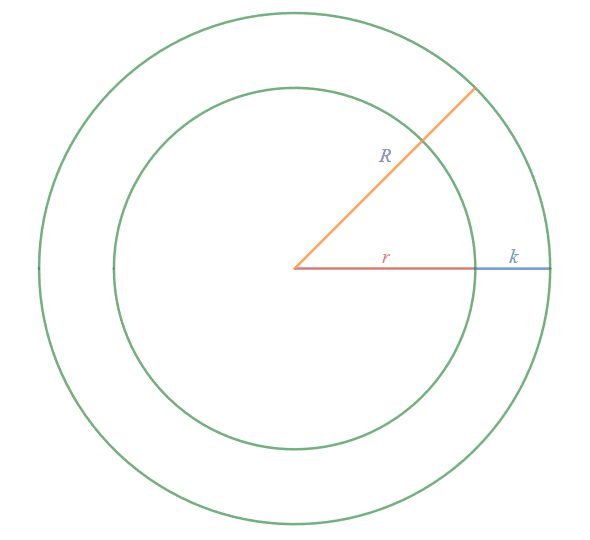# Relationship between inner and outer radius of a two concentric circles

• MHB
otlconcepts
If i have two circles that say 24" apart from each other. one inside the other.
and i know the radius of the inside circel, how can i calculate the outside radius

Joppy
MHB
Did you draw a picture?

[TIKZ]
\draw[thick,red] (0,0) circle (4);
\draw[->,thick,red] (0,0) -- (4, 0) node[below, xshift=-1cm] {$r_2$};
\draw[thick,blue] (0,0) circle (2);
\draw[->, thick, blue] (0,0) -- (2, 0) node[below, xshift=-0.75cm] {$r_1$};
[/TIKZ]

$r_2 - r_1 = 24"$ right? And you know $r_1$.

Last edited:
Gold Member
MHB
Hello, and welcome to MHB! :)

Suppose $$r$$ is the radius of the inner circle and $$R$$ is the radius of the inner circle, where $$k$$ is the difference between the two radii:

$$\displaystyle R-r=k$$